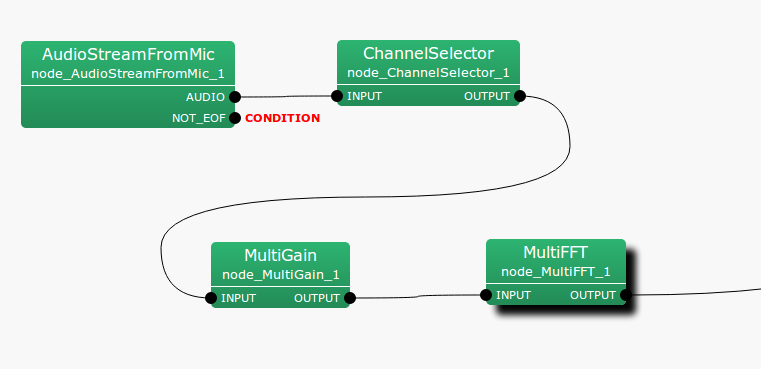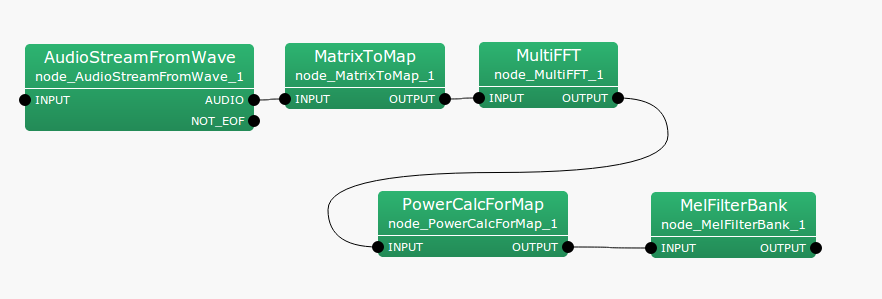## 6.7.22 MultiGain

### 6.7.22.1 Outline of the node

This node regulates gains of input signals.

### 6.7.22.2 Necessary files

No files are required.

### 6.7.22.3 Usage

When to use

This node is used to amplify input signals or modify them so that they cannot be clipped. For example, when speech waveform data quantified by 24 bits are used for inputs on a system built for 16 bits, the MultiGain node is used to decrease the gains by 8 bits.

Typical connection

This node is usually positioned just after AudioStreamFromMic or AudioStreamFromWave , or with ChannelSelector in between.Figure 6.118: Example of a connection of MultiGain

### 6.7.22.4 Input-output and properties of the node

Table 6.116: Parameters of MultiGain
 Parameter name Type Default value Unit Description GAIN 1.0 Gain value

Input

INPUT

: Matrix<float> type. Multichannel speech waveform data (time domain waveform).

Output

OUTPUT

: Matrix<float> type. Multichannel speech waveform data (time domain waveform) for which gains are regulated.

Parameters

GAIN

: float type. A gain parameter. Inputting 1.0 corresponds to outputting the inputs without change.

### 6.7.22.5 Details of the node

Each channel of inputs is output as the values multiplied by the value designated for the GAIN parameter. Note that the inputs are time domain waveforms. For example, when decreasing gains by 40 dB, calculate as follows and designate 0.01.

 $\displaystyle 20 \log x$ $\displaystyle =$ $\displaystyle -40$ (169) $\displaystyle x$ $\displaystyle =$ $\displaystyle 0.01$ (170)

subsectionPowerCalcForMap

### 6.7.22.6 Outline of the node

This node converts multichannel complex spectra of Map<int, ObjectRef> type IDs into real power/amplitude spectra.

### 6.7.22.7 Necessary files

No files are required.

### 6.7.22.8 Usage

When to use

This node is used to convert complex spectra into real power/amplitude spectra when inputs are Map<int, ObjectRef> type. When inputs are of Matrix<complex<float> > type, use the PowerCalcForMatrix node.

Typical connection

Figure 6.119 shows an example of the usage of the PowerCalcForMap node. A Map<int, ObjectRef> type complex spectrum obtained from the MultiFFT node is converted into Map<int, ObjectRef> type power spectrum and input to the MelFilterBank node.Figure 6.119: Example of a connection of PowerCalcForMap

### 6.7.22.9 Input-output and properties of the node

Table 6.117: Parameters of PowerCalcForMap
 Parameter name Type Default value Unit Description POWER_TYPE POW Selection of power/amplitude spectra

Input

INPUT

: Map<int, ObjectRef> type. Complex matrices of Matrix<complex<float> > type are stored in the ObjectRef part.

Output

OUTPUT

: Map<int, ObjectRef> type. Real matrices of power/absolute values are stored in the ObjectRef part for each element of the complex matrices of the inputs.

Parameter

POWER_TYPE

: string type. Selection of power (POW) or amplitude (MAG) spectra for the output.

### 6.7.22.10 Details of the node

The real matrix $N_{i,j}$ of the output for the complex matrix $M_{i,j}$ of an input ($i, j$ indicating the rows and columns of the index, respectively) is obtained as:

 $\displaystyle N_{i,j}$ $\displaystyle =$ $\displaystyle M_{i,j}M_{i,j}^{*}~ ~ (\textrm{if POWER\_ TYPE=POW}),$ $\displaystyle N_{i,j}$ $\displaystyle =$ $\displaystyle abs(M_{i,j})~ ~ (\textrm{if POWER\_ TYPE=MAG}),$

Here, $M_{i,j}^{*}$ indicates the complex conjugate of $M_{i,j}$.